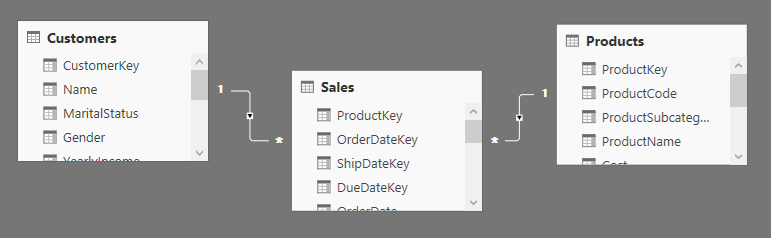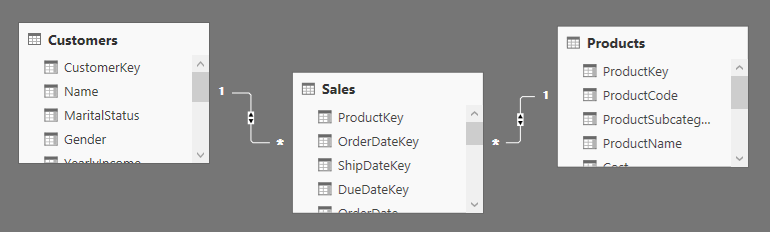## Row Context

DAX expressions operate on columns. You would usually write expressions like:

```Gross Profit := SUMX ( Sales, Sales[Amount] – Sales[TotalCost] )
```

In the previous expression, Sales[Amount] and Sales[TotalCost] are column references. A column reference intuitively means that you want to retrieve the value of a column. In the example above you would obtain the value of Sales[Amount] for the row currently iterated by SUMX.

In addition you can use a column reference to instruct certain functions to perform an action on a column, like in the following measure that counts the number of product names:

```NumOfAllProducts := COUNTROWS ( VALUES ( Product[ProductName] ) )
```

In this case, Product[ProductName] does not retrieve the value of ProductName for a specific row. Instead, you use the column reference to tell VALUES which column to use. In other words, you reference the column, not its value.

A column reference is a somewhat ambiguous definition because you reference the value of a column in a specific row and the full column itself, both with the same syntax. Nevertheless, DAX expressions are typically easy to read because, although ambiguous, the syntax leads to very intuitive expressions.

When you use a column reference to retrieve the value of a column in a given row, you need a way to tell DAX which row to use, out of the table, to compute the value. In other words, you need a way to define the current row of a table. This concept of “current row” defines the Row Context.

You have a row context whenever you iterate a table, either explicitly (using an iterator) or implicitly (in a calculated column):

• When you write an expression in a calculated column, the expression is evaluated for each row of the table, creating a row context for each row.
• When you use an iterator like FILTER, SUMX, AVERAGEX, ADDCOLUMNS, or any one of the DAX functions that iterate over a table expression.

If a row context is not available, evaluating a column reference produces an error. If you only write a column reference in a DAX measure, you get an error because no row context exists. For example, this measure is not valid:

```SalesAmount := Sales[Amount]
```

In order to make it work, you need to aggregate the column, not to refer to its value. In fact, the correct definition of SalesAmount is:

```SalesAmount := SUM ( Sales[Amount]  )
```

Every iterator introduces a new row context, and iterators can be nested. For example, you can write:

```AverageDiscountedSalesPerCustomer :=
AVERAGEX (
Customer,
SUMX (
RELATEDTABLE ( Sales ),
Sales[SalesAmount] * Customer[DiscountPct]
)
)
```

In the innermost expression, you reference both Sales[SalesAmount] and Customer[DiscountPct], i.e. two columns coming from different tables. You can safely do this because there are two row contexts: the first one introduced by AVERAGEX over Customer and the second one introduced by SUMX over Sales. Moreover, it is worth noting that the row context is also used by RELATEDTABLE to determine the set of rows to return. In fact, RELATEDTABLE ( Sales ) returns the sales of the current customer — by “current” we mean the customer currently iterated by AVERAGEX or, for a more clear definition, the current customer in the row context introduced by AVERAGEX over Customer.

## Filter Context

The filter context is the set of filters applied to the data model before the evaluation of a DAX expression starts. When you use a measure in a pivot table, for example, it produces different results for each cell because the same expression is evaluated over a different subset of the data. The Microsoft documentation describes as “query context” the filters applied by the user interface of a pivot table and as “filter context” the filters applied by DAX expressions that you can write in a measure. In reality, these filters are almost identical in their effects and the real differences are not important for this introductory article. We simply define as “filter context” the set of filters applied to the evaluation of a DAX expression — usually a measure — regardless of how they have been generated.

For example, the cell highlighted in the following picture has a filter context for year 2007, color equal to Black, and product brand equal to Contoso. This is the reason why its value is different, for example, from the one showing the same year for Fabrikam.You can obtain the same effect by applying a filter with CALCULATE or CALCULATETABLE. For example, the following DAX query returns the same value as that of the highlighted cell in the previous picture.

```EVALUATE
ROW (
"Sales Amount",
CALCULATE (
[Sales Amount],
'Date'[Calendar Year] = "CY 2007",
Product[Color] = "Black",
Product[Brand] = "Contoso"
)
)
```

Usually, every cell of a report has a different filter context, which can be defined implicitly by the user interface (such as the pivot table in Excel), or explicitly by a DAX expression using CALCULATE or CALCULATETABLE.

Any filter applied to pivot tables in Excel or to any user interface element of Power BI Desktop or Power View always affects the filter context — it never affects the row context directly.

A filter context is a set of filters over the rows of the data model. There is always a filter context for DAX expressions. If the filter context is empty, a DAX expression can iterate all the rows of the tables in a data model. When a filter context is not empty, it limits the rows that a DAX expression can iterate in a data model.

## Propagation of Filters

A row context does not propagate through relationships. If you have a row context in a table, you can iterate the rows of a table on the many side of a relationship using RELATEDTABLE, and you can access the row of a parent table using RELATED.

A filter applied on a table column affects all the rows of that table, filtering rows that satisfy that filter. If two or more filters are applied to columns in the same table, they are considered under a logical AND condition and only the rows satisfying all the filters are processed by a DAX expression in that filter context.

A filter context does automatically propagate through relationships, according to the cross filter direction of the relationship.

In Power Pivot for Excel, the only direction is one-to-many. A filter applied on the “one” side of a relationship affects the rows of the table on the “many” side of that relationship, but the filter does not propagate in the opposite direction. For example, consider a model where you have two tables, Product and Customer, each with a one-to-many relationship to the Sales table. With a single direction of cross filter, if you filter a product you also filter the sales of that product, but you do not filter the customer who bought those products.In Power BI Desktop and in SQL Server Analysis Services Tabular 2016, you can enable bidirectional cross filtering. By enabling the bidirectional cross filter, once you filter one table, you also filter all the tables on the “one” side of a relationship. For example, when you filter rows on Product, you implicitly filter Sales and Customer, thus filtering customers who bought the selected products.When writing DAX expressions, you can control both the row context and the filter context. Remember that the row context does not propagate automatically through relationships, whereas the filter context does traverse relationships independently from the DAX code. However, you can control the filter context and its propagation using DAX functions such as CALCULATE, CALCULATETABLE, ALL, VALUES, FILTER, USERELATIONSHIP, and CROSSFILTER.

SUMXReturns the sum of an expression evaluated for each row in a table.

`SUMX ( <Table>, <Expression> )`

VALUESWhen a column name is given, returns a single column table of unique values. When a table name is given, returns a table with the same columns and all the rows of the table (including duplicates) with the additional blank row if present.

`VALUES ( <TableNameOrColumnName> )`

FILTERReturns a table that has been filtered.

`FILTER ( <Table>, <FilterExpression> )`

AVERAGEXCalculates the average (arithmetic mean) of a set of expressions evaluated over a table.

`AVERAGEX ( <Table>, <Expression> )`

ADDCOLUMNSReturns a table with new columns specified by the DAX expressions.

`ADDCOLUMNS ( <Table>, <Name>, <Expression> [, <Name>, <Expression> [, … ] ] )`

RELATEDTABLEContext transition

Returns the related tables filtered so that it only includes the related rows.

`RELATEDTABLE ( <Table> )`

CALCULATEContext transition

Evaluates an expression in a context modified by filters.

`CALCULATE ( <Expression> [, <Filter> [, <Filter> [, … ] ] ] )`

CALCULATETABLEContext transition

Evaluates a table expression in a context modified by filters.

`CALCULATETABLE ( <Table> [, <Filter> [, <Filter> [, … ] ] ] )`

ANDChecks whether all arguments are TRUE, and returns TRUE if all arguments are TRUE.

`AND ( <Logical1>, <Logical2> )`

ALLCALCULATE modifier

Returns all the rows in a table, or all the values in a column, ignoring any filters that might have been applied.

`ALL ( [<TableNameOrColumnName>] [, <ColumnName> [, <ColumnName> [, … ] ] ] )`

USERELATIONSHIPCALCULATE modifier

Specifies an existing relationship to be used in the evaluation of a DAX expression. The relationship is defined by naming, as arguments, the two columns that serve as endpoints.

`USERELATIONSHIP ( <ColumnName1>, <ColumnName2> )`

CROSSFILTERCALCULATE modifier

Specifies cross filtering direction to be used in the evaluation of a DAX expression. The relationship is defined by naming, as arguments, the two columns that serve as endpoints.

`CROSSFILTER ( <LeftColumnName>, <RightColumnName>, <CrossFilterType> )`